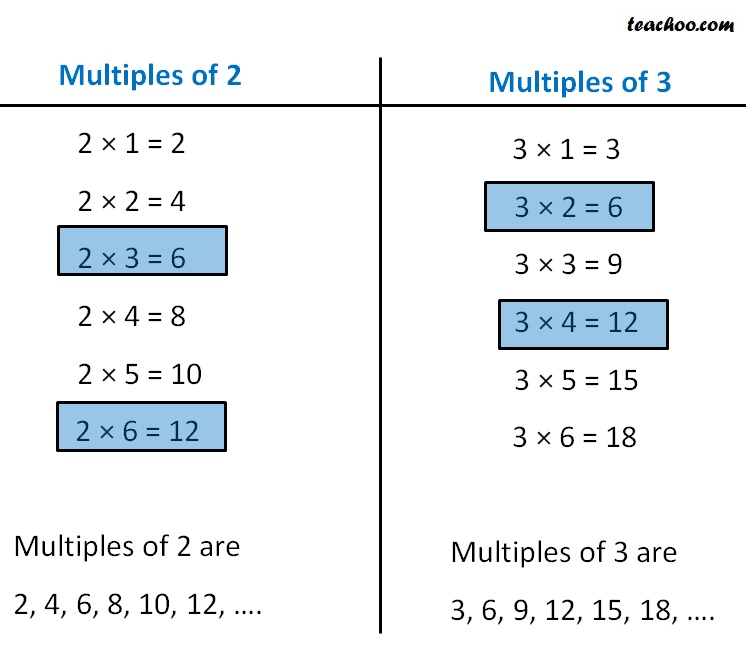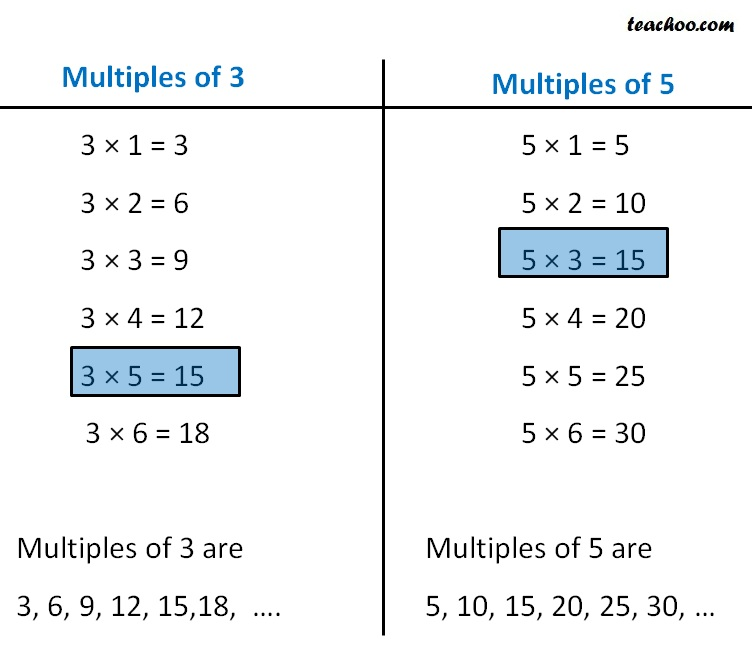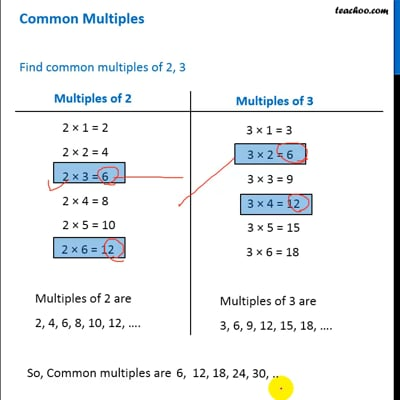Common Multiples

Chapter 3 Class 6 Playing with Numbers
Concept wise

## Find common multiples of 2, 3So, Common multiples are 6, 12, 18, 24, 30

## Find common multiples of 3, 5So, Common multiples are 15, 30, 45, 60, 75This video is only available for Teachoo black users

### Transcript

Multiples of 2 2 × 1 = 2 2 × 2 = 4 2 × 3 = 6 2 × 4 = 8 2 × 5 = 10 2 × 6 = 12 Multiples of 2 are 2, 4, 6, 8, 10, 12, …. Multiples of 3 3 × 1 = 3 3 × 2 = 6 3 × 3 = 9 3 × 4 = 12 3 × 5 = 15 3 × 6 = 18 Multiples of 3 are 3, 6, 9, 12, 15, 18, …. Multiples of 3 3 × 1 = 3 3 × 2 = 6 3 × 3 = 9 3 × 4 = 12 3 × 5 = 15 3 × 6 = 18 Multiples of 3 are 3, 6, 9, 12, 15,18, …. Multiples of 5 5 × 1 = 5 5 × 2 = 10 5 × 3 = 15 5 × 4 = 20 5 × 5 = 25 5 × 6 = 30 Multiples of 5 are 5, 10, 15, 20, 25, 30, …